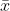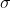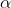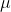# Two hundred fish caught in Cay

Two hundred fish caught in Cayuga Lake had a mean length of 13.8inches. The population standard deviation is 3.8 inches. (Give youranswer correct to two decimal places.)

(a) Find the 90% confidence interval for the population meanlength.

 Lower Limit Upper Limit

(b) Find the 98% confidence interval for the population meanlength.

 Lower Limit Upper Limit

Solution:-

Given that,= 13.8= 3.8

n = 200

A ) At 90% confidence level the z is ,=1 – 90% = 1 – 0.90 = 0.10/ 2 = 0.10 / 2 = 0.05

Z/2= Z0.05 = 1.645

Margin of error = E = Z/2*(/n)

= 1.645 * (3.8 / 200 ) = 0.442

At 95% confidence interval estimate of the population meanis,– E <<+ E

13.8 – 0.442<< 13.8  + 0.442

13.36 <<14.24

(13.36 , 14.24)

Lower limit – 13.36

Upper limit – 14.24

b) At 98% confidence level the z is ,=1 – 98% = 1 – 0.98 = 0.02/ 2 = 0.02 / 2 = 0.01

Z/2= Z0.01 = 2.326

Margin of error = E = Z/2*(/n)

= 1.326 * (3.8 / 200) = 0.625

At 98% confidence interval estimate of the population meanis,– E <<+ E

13.8 – 0.625 << 13.8 + 0.625

13.18 << 14.42

(13.18 , 14.42)

Lower limit – 13.18

Upper limit – 14.42

##### "Our Prices Start at \$11.99. As Our First Client, Use Coupon Code GET15 to claim 15% Discount This Month!!"Pages (275 words)
Standard price: \$0.00
Client Reviews
4.9
Sitejabber
4.6
Trustpilot
4.8
Our Guarantees
100% Confidentiality
Information about customers is confidential and never disclosed to third parties.
Original Writing
We complete all papers from scratch. You can get a plagiarism report.
Timely Delivery
No missed deadlines – 97% of assignments are completed in time.
Money Back